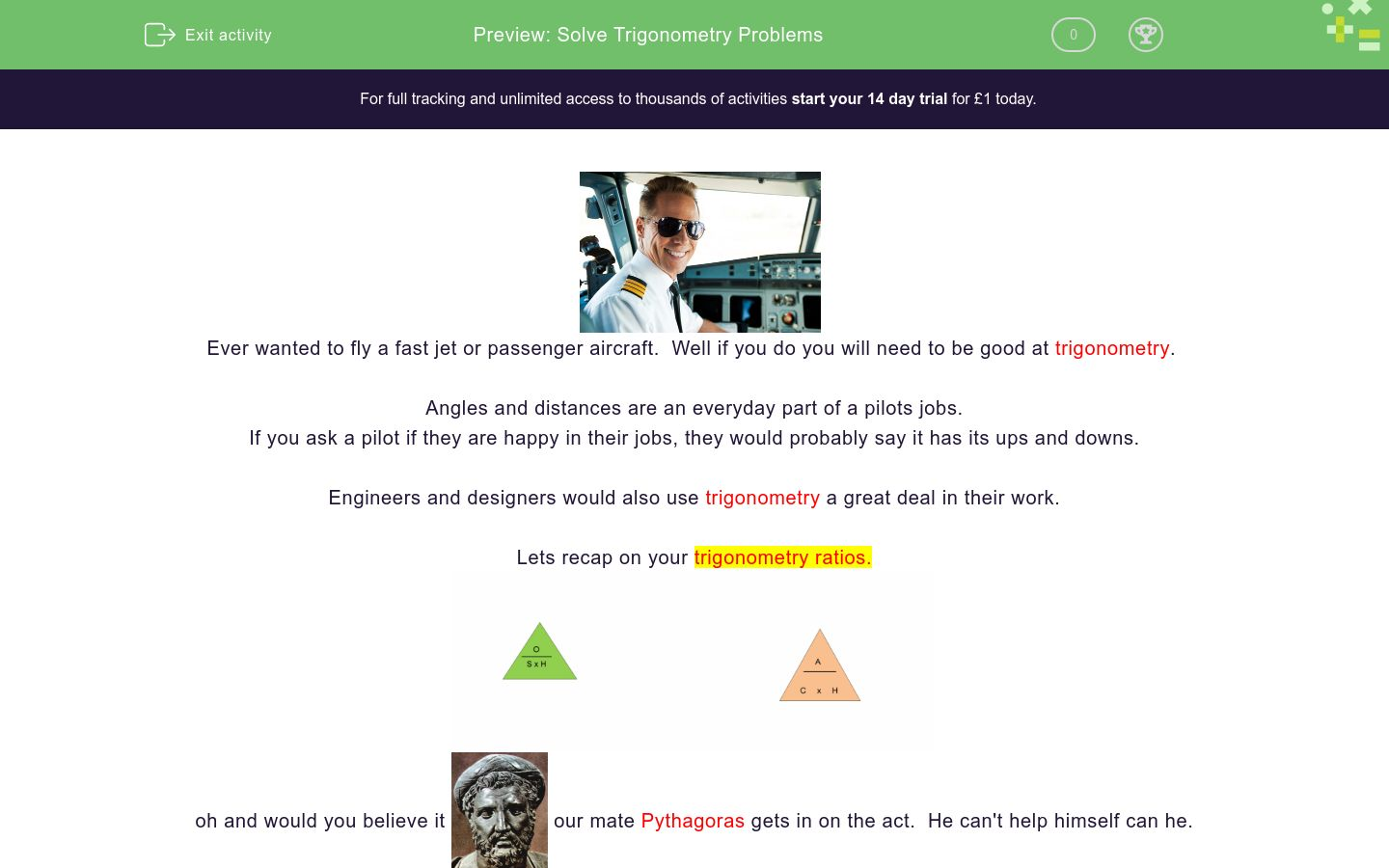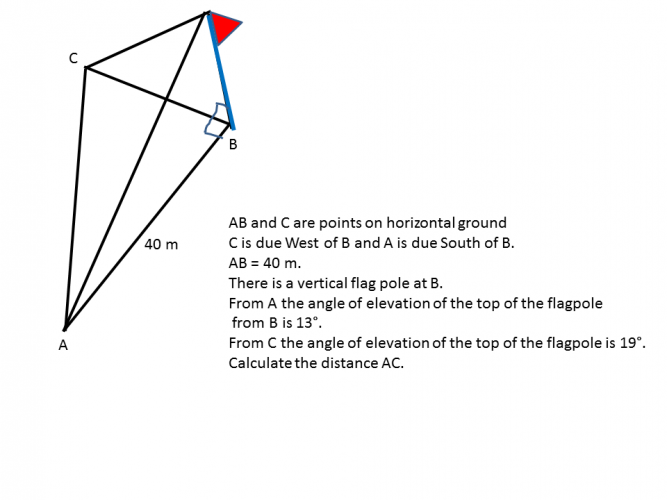# Solve Trigonometry Problems

In this worksheet, students will apply Pythagoras' Theorem and trigonometry within 3D shapes. A requirement of higher level maths is to apply more than one skill within the same question.Key stage:  KS 4

GCSE Subjects:   Maths

GCSE Boards:   AQA, Eduqas, OCR, Pearson Edexcel

Curriculum topic:   Geometry and Measures, Mensuration

Curriculum subtopic:   Mensuration and Calculation, Triangle Mensuration

Difficulty level:### QUESTION 1 of 10Ever wanted to fly a fast jet or passenger aircraft.  Well if you do you will need to be good at trigonometry

Angles and distances are an everyday part of a pilots jobs.

If you ask a pilot if they are happy in their jobs, they would probably say it has its ups and downs.

Engineers and designers would also use trigonometry a great deal in their work.

Lets recap on your trigonometry ratios.oh and would you believe itour mate Pythagoras gets in on the act.  He can't help himself can he.

Pythagoras' Theorem

a² + b² = c²

I wonder if pilots ever think about him when the fly over Greece.

The trig ratios are often combined with Pythagoras's Theorem when solving problems relating to right angled triangles.

Lets have a goFigure 1 just asks the question, that may well be all that you are given.

Figure 2 Draw in the right angled triangle.

Figure 3 Mark in the angle.  Remember it is the middle letter A.

Pythagoras' Theorem has also been applied in figure 3 to find the length of the diagonal.  We need this before we can calculate the angle.

30 cm² + 10 cm² = √1000 = 31.622..... cm

Mark this on the diagram to help see the trig ratio that you need.

20 ÷ 31.6222 = 0.63246...... tan-1 0.63246 = 32.3° correct to 1 decimal place.

There is quite a lot to take in here so take it one step at a time.

Here is a cuboid. AB = 6 cm BC = 8 cm and AE = 3 cm

Find angle ECA14.5°

18.3°

15.2°

16.7°

Here is a cuboid.

AB = 12 cm BC = 5 cm and AE = 4 cm.

Find angle GAC13.6° 17.1° 16.3° 14.7° Angle GAC is

AB = 6 cm BC = 8 cm and CG = 18 cm

Here is a cuboid.Here is a cube with side 5 cm.29.7°

42.2°

32.4°

27.8°78.0° 54.6° 65.4° 72.6° The angle is78.0° 54.6° 65.4° 72.6° The angle is• Question 1

Here is a cuboid. AB = 6 cm BC = 8 cm and AE = 3 cm

Find angle ECA16.7°
EDDIE SAYS
Did you spot and draw in the right angled triangle okay? Once you have found it things should become clearer and more straightforward, just as we like it.• Question 2

Here is a cuboid.

AB = 12 cm BC = 5 cm and AE = 4 cm.

Find angle GAC13.6° 17.1° 16.3° 14.7° Angle GAC is
EDDIE SAYS
Good old Pythagoras', he is well worth knowing isn't he? He helps to break the problem down into manageable chunks.• Question 3

AB = 6 cm BC = 8 cm and CG = 18 cm

Here is a cuboid.29.1
EDDIE SAYS
How did you find this one?• Question 4Here is a cube with side 5 cm.

EDDIE SAYS
Did your remember that a cube has all sides the same? This should make things a little easier with Pythagoras' Theorem.• Question 527.8°
EDDIE SAYS
Ooh, this is an interesting shape. As long as you can spot the right angled triangles you will be okay.• Question 640.1
EDDIE SAYS
Are you finding this easier now? Always draw the triangles, it is to much to try and visualise them and then recall Pythagoras' Theorem and trigonometry!!• Question 778.0° 54.6° 65.4° 72.6° The angle is
EDDIE SAYS
Just when you thought everything was okay, another shape is thrown in. It is just more practice so that you can work with any 3d shape.• Question 8EDDIE SAYS
Are you getting the hang of this now. The more you practice the easier it becomes.• Question 917.2
EDDIE SAYS
Oh yes even more ways to present a 3D trigonometry problem. This is an example of how trigonometry may be used in the real world. Engineers would use this. Are you able to give them any tips?• Question 1048.1
EDDIE SAYS
Oh really I hear you say. This is quite straightforward if a little work. Well you had to finish on a flourish.---- OR ----

Sign up for a £1 trial so you can track and measure your child's progress on this activity.

### What is EdPlace?

We're your National Curriculum aligned online education content provider helping each child succeed in English, maths and science from year 1 to GCSE. With an EdPlace account you’ll be able to track and measure progress, helping each child achieve their best. We build confidence and attainment by personalising each child’s learning at a level that suits them.

Get started